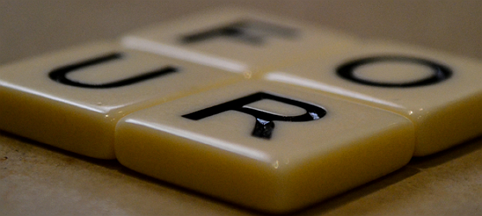# SuperSquaresA supersquare is a 4 digit number $\overline{ABCD}$ such as the two digit numbers $\overline{AB}, \overline{BC},\overline{CD}$ are all perfect squares.

What is the sum of all supersquare(s)?